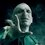# Derivative confusion

$x\in \Re$ $\\ f\left( x \right) =x+x+x+......x\quad \quad (x\quad times)\$/extract_itex] $Then\quad first\quad derivative\quad of\quad f\left( x \right) \quad will\quad be\quad x\quad or\quad 2x?$ The confusion arrived due to a problem similar to it.Note by Abhijeet Verma 6 years, 3 months ago This discussion board is a place to discuss our Daily Challenges and the math and science related to those challenges. Explanations are more than just a solution — they should explain the steps and thinking strategies that you used to obtain the solution. Comments should further the discussion of math and science. When posting on Brilliant: • Use the emojis to react to an explanation, whether you're congratulating a job well done , or just really confused . • Ask specific questions about the challenge or the steps in somebody's explanation. Well-posed questions can add a lot to the discussion, but posting "I don't understand!" doesn't help anyone. • Try to contribute something new to the discussion, whether it is an extension, generalization or other idea related to the challenge. • Stay on topic — we're all here to learn more about math and science, not to hear about your favorite get-rich-quick scheme or current world events. MarkdownAppears as *italics* or _italics_ italics **bold** or __bold__ bold - bulleted- list • bulleted • list 1. numbered2. list 1. numbered 2. list Note: you must add a full line of space before and after lists for them to show up correctly paragraph 1paragraph 2 paragraph 1 paragraph 2 [example link](https://brilliant.org)example link > This is a quote This is a quote  # I indented these lines # 4 spaces, and now they show # up as a code block. print "hello world" # I indented these lines # 4 spaces, and now they show # up as a code block. print "hello world" MathAppears as Remember to wrap math in $$ ... $$ or \[ ... $ to ensure proper formatting.
2 \times 3 $2 \times 3$
2^{34} $2^{34}$
a_{i-1} $a_{i-1}$
\frac{2}{3} $\frac{2}{3}$
\sqrt{2} $\sqrt{2}$
\sum_{i=1}^3 $\sum_{i=1}^3$
\sin \theta $\sin \theta$
\boxed{123} $\boxed{123}$

Sort by:

- 6 years, 3 months ago

Is $x$ an integer? If not then the expression $x^2 = x+ x+ \dots \dots x \text{ times }$ doesn't make sense.

- 6 years, 3 months ago

If we don't restrict x to integers ,then ? (Addition of continuous functions should yield a continuous function )

- 6 years, 3 months ago

If x is an integer then function won't be continuous hence Differentiating it would be meaningless.

- 6 years, 3 months ago

But x doesn't only belong to integers. $x\quad \in \quad \Re$

- 6 years, 3 months ago

Try writing down the function for $x = 2.5$.

- 6 years, 3 months ago

Then how can you write $x^2$ 'x' times x when x is not integer?

- 6 years, 3 months ago

Yeah , adding x times x itself implies that x has to be a whole number. Thus its differentiation would have no meaning.Thanks and sry

- 6 years, 3 months ago

y=x is a continuous function. Thus, y=x+x+x+....x will also be a continuous function for all x belongs to real.

- 6 years, 3 months ago